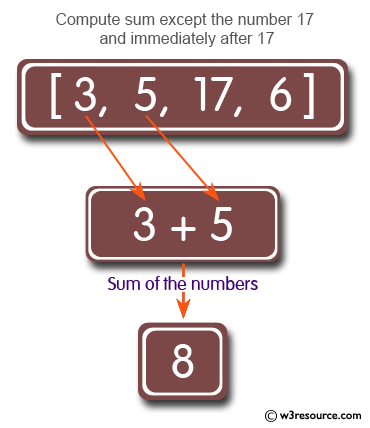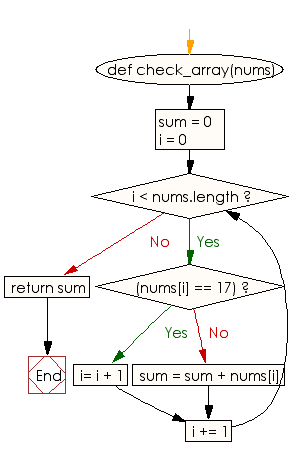﻿ Ruby Array exercises: Compute the sum of the numbers of a given array except the number 17 and numbers that come immediately after a 17 - w3resource# Ruby Array Exercises: Compute the sum of the numbers of a given array except the number 17 and numbers that come immediately after a 17

## Ruby Array: Exercise-32 with Solution

Write a Ruby program to compute the sum of the numbers of a given array except the number 17 and numbers that come immediately after a 17. Return 0 for an empty array.Ruby Code:

``````def check_array(nums)
sum = 0
i = 0
while i < nums.length
if(nums[i] == 17)
i= i + 1
else
sum = sum + nums[i]
end
i += 1
end
return sum
end
print check_array([3, 5, 17, 6]),"\n"
print check_array([3, 5, 1, 17]),"\n"
print check_array([3, 17, 1, 7]),"\n"
``````

Output:

```8
9
10
```

Flowchart:Ruby Code Editor: